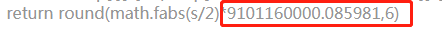import math
def ComputeArea(data):
arr = data.split(';')
arr_len = len(arr)
if arr_len < 3:
return 0.0
temp = []
for i in range(0,arr_len):
temp.append([float(x) for x in arr[i].split(',')])
s = temp * (temp[arr_len -1]-temp)
print s
for i in range(1,arr_len):
s += temp[i] * (temp[i-1] - temp[(i+1)%arr_len])
return round(math.fabs(s/2)*9101160000.085981,6)

2个回答arcgis10利用经纬度计算多边形面积

30.5948166666666 122.1237166666660 30.5943666666666 122.1201500000000 30.5942833333333 122.1199666666660 30.5940833333333 122.1181833333330 30.5938666666666 122.1174166666660 30.5937333333333 122.1172833333330 30.5921833333333 122.1183333333330 30.5913833333333 122.1200333333330 30.5910333333333 122.1210000000000 30.5910666666666 122.1213166666660 30.5914500000000 122.1219166666660 30.5917500000000 122.1226833333330 30.5918166666666 122.1232666666660 30.5925000000000 122.1221000000000 30.5929333333333 122.1216666666660 30.5933500000000 122.1213500000000 30.5939000000000 122.1215333333330 30.5942333333333 122.1218333333330 30.5947333333333 122.1236333333330 经纬度数据如图，没有shape文件，请热心网友们告诉我怎么算这个面积

arcgis利用经纬度计算多边形面积

java 经纬度计算距离 经纬和距离切换

redis做缓存之后怎么根据经纬度查询数据

![图片说明](https://img-ask.csdn.net/upload/201911/21/1574350838_130711.jpg) 如何在表格添加一列距离 表示上下两行经纬度距离？ 这是我的代码 ``` import pandas as pd import numpy as np gps1=pd.read_csv('top_01.csv',sep=',') truck1029943=gps1[gps1['user_id']=='1029943'] #按时间升序排列 truck11029943=truck1029943.sort_values(by='time', ascending=True) #根据经纬度坐标计算直线距离 from math import radians, cos, sin, asin, sqrt def haversine(lon1, lat1, lon2, lat2): # 经度1，纬度1，经度2，纬度2 （十进制度数） lon1, lat1, lon2, lat2 = map(radians, [lon1, lat1, lon2, lat2]) # 将十进制度数转化为弧度 dlon = lon2 - lon1 dlat = lat2 - lat1 a = sin(dlat/2)**2 + cos(lat1) * cos(lat2) * sin(dlon/2)**2 c = 2 * asin(sqrt(a)) r = 6371 # 地球平均半径，单位为公里 return c * r * 1000 #list(map函数) truck1029943.loc[truck1029943['latitude']>0,'distance']=list(map(haversine, truck1029943['longitude'], truck1029943['latitude'], truck1029943['longitude'].shift(1), truck1029943['latitude'].shift(1)));truck1029943 #pd.set_option('display.max_columns',1000) print(truck1029943) ``` 不知道哪里错误了 请指正，想输出的表格直接在最后是有距离的

SQLSERVER函数，算两个经纬度间的距离

SQLSERVER函数，算两个经纬度间的距离 CREATE FUNCTION getDistatce (@relat double,@p_lat double,@relng double,@p_lng double) returns double AS BEGIN DECLARE R double,distance double,dLat double,dLon double,a double; SET R = 6371.0,distance = 0.0,dLat = (@relat - @p_lat)*PI() /180,dLon = (@relng - @p_lng)*PI() /180; SET a = SIN(dLat/2)*SIN(dLat/2) + COS(@p_lat*PI()/180) *COS(@relat*PI()/180) *sin(dLon/2)*SIN(dLon/2); SET distance = floor((2*ATAN(SQRT(a),SQRT(1-a)))*R*1000); RETURN distance; END GO 这段函数是出错的 ，求大神修正或重新写一个函数 感谢各位

LBS方案中的经纬度距离计算

Java校招入职华为，半年后我跑路了

Java基础知识面试题（2020最新版）

@程序员：GitHub这个项目快薅羊毛

C++(继承):19---虚基类与虚继承（virtual）

loonggg读完需要3分钟速读仅需 1 分钟大家好，我是你们的校长。我之前讲过，这年头，只要肯动脑，肯行动，程序员凭借自己的技术，赚钱的方式还是有很多种的。仅仅靠在公司出卖自己的劳动时...

win10暴力查看wifi密码

MySQL数据库面试题（2020最新版）

!大部分程序员只会写3年代码

2020阿里全球数学大赛：3万名高手、4道题、2天2夜未交卷